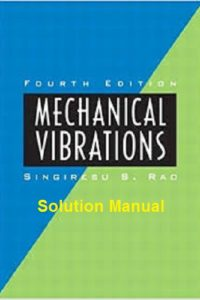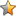اسم المؤلف
Dr. Singiresu S. Rao
التاريخ
1 مايو 2018
المشاهدات
التقييمLoading...

حل كتاب
Mechanical Vibrations 4th Edition Solution Manual
Dr. Singiresu S. Rao
CHAPTER
Fundamentals of Vibration
Preliminary Remarks
Brief History of the Study of Vibration
Origins of the Study of Vibration
From Galileo to Rayleigh
Recent Contributions
Importance of the Study of Vibration
Basic Concepts of Vibration
Vibration
Elementary Parts of
Vibrating Systems
Number of Degrees of Freedom
Discrete and Continuous Systems
Classification of Vibration
Free and Forced Vibration
Undamped and Damped Vibration
Linear and Nonlinear Vibration
Deterministic and
Random Vibration
Vibration Analysis Procedure
Spring Elements
Nonlinear Springs
Linearization of a
Nonlinear Spring
Spring Constants of Elastic Elements
Combination of Springs
Spring Constant Associated with the
Restoring Force due to Gravity
Mass or Inertia Elements
Combination of Masses
Damping Elements
Construction of Viscous Dampers
Linearization of a
Nonlinear Damper
Combination of Dampers
Harmonic Motion
Vectorial Representation of
Harmonic Motion
Complex-Number Representation
of Harmonic Motion
Complex Algebra
Operations on Harmonic Functions
Definitions and Terminology
Harmonic Analysis
Fourier Series Expansion
Complex Fourier Series
Frequency Spectrum
Time- and Frequency-Domain
Representations
Even and Odd Functions
Half-Range Expansions
Numerical Computation
of Coefficients
Examples Using MATLAB
Vibration Literature
Chapter Summary
References
Review Questions
Problems
Design Projects
CONTENTS v
CHAPTER
Free Vibration of Single-Degree-of-Freedom
Systems
Introduction
Free Vibration of an Undamped
Translational System
Equation of Motion Using Newton’s
Second Law of Motion
Equation of Motion Using Other
Methods
Equation of Motion of a Spring-Mass
System in Vertical Position
Solution
Harmonic Motion
Free Vibration of an Undamped
Torsional System
Equation of Motion
Solution
Response of First Order Systems
and Time Constant
Rayleigh’s Energy Method
Free Vibration with Viscous Damping
Equation of Motion
Solution
Logarithmic Decrement
Energy Dissipated in Viscous
Damping
Torsional Systems with Viscous
Damping
Graphical Representation of Characteristic Roots
and Corresponding Solutions
Roots of the Characteristic Equation
Graphical Representation of Roots and
Corresponding Solutions
Parameter Variations and Root Locus
Representations
Interpretations of and
in s-plane
Root Locus and Parameter
Variations
Free Vibration with Coulomb Damping
Equation of Motion
Solution
Torsional Systems with Coulomb
Damping
Free Vibration with Hysteretic Damping
Stability of Systems
Examples Using MATLAB
Chapter Summary
References
Review Questions
Problems
Design Projects
CHAPTER
Harmonically Excited Vibration
Introduction
Equation of Motion
Response of an Undamped System
Under Harmonic Force
Total Response
Beating Phenomenon
Response of a Damped System Under
Harmonic Force
Total Response
Quality Factor and Bandwidth
Response of a Damped System
Under
Response of a Damped System Under the
Harmonic Motion of the Base
Force Transmitted
Relative Motion
Response of a Damped System Under Rotating
Unbalance
Forced Vibration with Coulomb Damping
Forced Vibration with Hysteresis Damping
Forced Motion with Other Types of
Damping
Self-Excitation and Stability Analysis
Dynamic Stability Analysis
Dynamic Instability Caused by Fluid
Flow
Transfer-Function Approach
Solutions Using Laplace Transforms
Frequency Transfer Functions
Relation Between the General Transfer
function T(s) and the Frequency Transfer
Function
Representation of Frequency-Response
Characteristics
T(iv)
F(t) =Fe
iVt
Examples Using MATLAB
Chapter Summary
References
Review Questions
Problems
Design Projects
CHAPTER
Vibration Under General Forcing
Conditions
Introduction
Response Under a General
Periodic Force
First-Order Systems
Second-Order Systems
Response Under a Periodic Force
of Irregular Form
Response Under a Nonperiodic Force
Convolution Integral
Response to an Impulse
Response to a General Forcing
Condition
Response to Base Excitation
Response Spectrum
Response Spectrum for Base
Excitation
Earthquake Response Spectra
Design Under a Shock
Environment
Laplace Transform
Responses
Response of First-Order Systems
Response of Second-Order Systems
Response to Step Force
Analysis of the Step Response
Description of Transient
Response
Numerical Methods
Runge-Kutta Methods
Response to Irregular Forcing Conditions Using
Numerical Methods
Examples Using MATLAB
Chapter Summary
References
Review Questions
Problems
Design Projects
CHAPTER
Two-Degree-of-Freedom Systems
Introduction
Equations of Motion for Forced
Vibration
Free Vibration Analysis of an Undamped
System
Torsional System
Coordinate Coupling and Principal
Coordinates
Forced-Vibration Analysis
Semidefinite Systems
Self-Excitation and Stability
Analysis
Transfer-Function Approach
Solutions Using Laplace Transform
Solutions Using Frequency Transfer
Functions
Examples Using MATLAB
Chapter Summary
References
Review Questions
Problems
Design Projects
CHAPTER
Multidegree-of-Freedom Systems
Introduction
Modeling of Continuous Systems as Multidegreeof-Freedom Systems
Using Newton’s Second Law to Derive Equations
of Motion
Influence Coefficients
Stiffness Influence Coefficients
Flexibility Influence Coefficients
Inertia Influence Coefficients
Potential and Kinetic Energy Expressions in
Matrix Form
Generalized Coordinates and Generalized
Forces
Using Lagrange’s Equations to Derive Equations
of Motion
Equations of Motion of Undamped Systems in
Matrix Form
Eigenvalue Problem
Solution of the Eigenvalue Problem
Solution of the Characteristic
(Polynomial) Equation
Orthogonality of Normal Modes
Repeated Eigenvalues
Expansion Theorem
Unrestrained Systems
Free Vibration of Undamped Systems
Forced Vibration of Undamped Systems Using
Modal Analysis
Forced Vibration of Viscously Damped
Systems
Self-Excitation and Stability Analysis
Examples Using MATLAB
Chapter Summary
References
Review Questions
Problems
Design Project
CHAPTER
Determination of Natural Frequencies and
Mode Shapes
Introduction
Dunkerley’s Formula
Rayleigh’s Method
Properties of Rayleigh’s Quotient
Computation of the Fundamental Natural
Frequency
Fundamental Frequency of Beams and
Shafts
Holzer’s Method
Torsional Systems
Spring-Mass Systems
Matrix Iteration Method
Convergence to the Highest Natural
Frequency
Computation of Intermediate Natural
Frequencies
Jacobi’s Method
Standard Eigenvalue Problem
Choleski Decomposition
Other Solution Methods
Examples Using MATLAB
Chapter Summary
References
Review Questions
Problems
Design Projects
CHAPTER
Continuous Systems
Introduction
Transverse Vibration of a String or
Cable
Equation of Motion
Initial and Boundary Conditions
Free Vibration of a Uniform
String
Free Vibration of a String with Both Ends
Fixed
Traveling-Wave Solution
Longitudinal Vibration of a Bar or Rod
Equation of Motion
and Solution
Orthogonality of Normal
Functions
Torsional Vibration of a Shaft or Rod
Lateral Vibration of Beams
Equation of Motion
Initial Conditions
Free Vibration
Boundary Conditions
Orthogonality of Normal
Functions
Forced Vibration
Effect of Axial Force
Effects of Rotary Inertia and Shear
Deformation
Other Effects
Vibration of Membranes
Equation of Motion
Initial and Boundary Conditions
Rayleigh’s Method
The Rayleigh-Ritz Method
Examples Using MATLAB
Chapter Summary
References
Review Questions
Problems
Design Project
CHAPTER
Vibration Control
Introduction
Vibration Nomograph and Vibration
Criteria
Reduction of Vibration at the Source
Balancing of Rotating Machines
Single-Plane Balancing
Two-Plane Balancing
Whirling of Rotating Shafts
Equations of Motion
Critical Speeds
Response of the System
Stability Analysis
Balancing of Reciprocating Engines
Unbalanced Forces Due to Fluctuations in
Gas Pressure
Unbalanced Forces Due to Inertia of the
Moving Parts
Balancing of Reciprocating
Engines
Control of Vibration
Control of Natural Frequencies
Introduction of Damping
Vibration Isolation
Vibration Isolation System with Rigid
Foundation
Vibration Isolation System with Base
Motion
Vibration Isolation System with Flexible
Foundation
Vibration Isolation System with Partially
Flexible Foundation
Shock Isolation
Active Vibration Control
Vibration Absorbers
Undamped Dynamic Vibration
Absorber
Damped Dynamic Vibration
Absorber
Examples Using MATLAB
Chapter Summary
References
Review Questions
Problems
Design Project
CHAPTER
Vibration Measurement and
Applications
Introduction
Transducers
Variable Resistance Transducers
Piezoelectric Transducers
Electrodynamic Transducers
Linear Variable Differential Transformer
Transducer
Vibration Pickups
Vibrometer
Accelerometer
Velometer
Phase Distortion
Frequency-Measuring Instruments
Vibration Exciters
Mechanical Exciters
Electrodynamic Shaker
Signal Analysis
Spectrum Analyzers
Bandpass Filter
Constant-Percent Bandwidth and
Constant-Bandwidth Analyzers
Dynamic Testing of Machines
and Structures
Using Operational Deflection-Shape
Measurements
Using Modal Testing
Experimental Modal Analysis
The Basic Idea
The Necessary Equipment
Digital Signal Processing
Analysis of Random Signals
Determination of Modal Data
from Observed Peaks
Determination of Modal Data
from Nyquist Plot
Measurement of Mode Shapes
Machine–Condition Monitoring
and Diagnosis
Vibration Severity Criteria
Machine Maintenance Techniques
Machine–Condition Monitoring
Techniques
Vibration Monitoring Techniques
Instrumentation Systems
Choice of Monitoring Parameter
Examples Using MATLAB
Chapter Summary
References
Review Questions
Problems
Design Projects
CHAPTER
Numerical Integration Methods in
Vibration Analysis
Introduction
Finite Difference Method
Central Difference Method for Single-Degree-ofFreedom Systems
Runge-Kutta Method for Single-Degree-ofFreedom Systems
Central Difference Method for Multidegree-ofFreedom Systems
Finite Difference Method for Continuous
Systems
Longitudinal Vibration of Bars
Transverse Vibration of Beams
Runge-Kutta Method for Multidegree-ofFreedom Systems
Houbolt Method
Wilson Method
Newmark Method
Examples Using MATLAB
Chapter Summary
References
Review Questions
Problems
CHAPTER
Finite Element Method
Introduction
Equations of Motion of an Element
Mass Matrix, Stiffness Matrix, and Force
Vector
Bar Element
Torsion Element
Beam Element
Transformation of Element Matrices and
Vectors
Equations of Motion of the Complete System of
Finite Elements
Incorporation of Boundary
Conditions
Consistent- and Lumped-Mass Matrices
Lumped-Mass Matrix for a Bar
Element
Lumped-Mass Matrix for a Beam
Element
Lumped-Mass Versus Consistent-Mass
Matrices
Examples Using MATLAB
Chapter Summary
References
Review Questions
Problems
Design Projects
files on the Companion Website
CHAPTER
Nonlinear Vibration –
Introduction –
Examples of Nonlinear Vibration Problems –
Simple Pendulum –
Mechanical Chatter, Belt Friction
System –
Variable Mass System –
Exact Methods –
Approximate Analytical Methods –
Basic Philosophy –
Lindstedt’s Perturbation Method –
Iterative Method –
Ritz-Galerkin Method –
Subharmonic and Superharmonic
Oscillations –
Subharmonic Oscillations –
Superharmonic Oscillations –
Systems with Time-Dependent Coefficients
(Mathieu Equation) –
Graphical Methods –
Phase-Plane Representation –
Phase Velocity –
Method of Constructing
Trajectories –
Obtaining Time Solution from Phase
Plane Trajectories –
Stability of Equilibrium States –
Stability Analysis –
Classification of Singular
Points –
Limit Cycles –
Chaos –
Functions with Stable Orbits –
Functions with Unstable Orbits –
Chaotic Behavior of Duffing’s Equation
Without the Forcing Term –
Chaotic Behavior of Duffing’s Equation
with the Forcing Term –
Numerical Methods –
Examples Using MATLAB –
Chapter Summary –
References –
Review Questions –
Problems –
Design Projects –
CHAPTER
Random Vibration –
Introduction –
Random Variables and Random Processes –
Probability Distribution –
Mean Value and Standard Deviation –
Joint Probability Distribution of Several
Random Variables –
Correlation Functions of a Random Process –
Stationary Random Process –
Gaussian Random Process –
Fourier Analysis –
Fourier Series –
Fourier Integral –
Power Spectral Density –
Wide-Band and Narrow-Band Processes –
Response of a Single-Degree-ofFreedom System –
Impulse-Response Approach –
Frequency-Response Approach –
Characteristics of the Response
Function –
Response Due to Stationary Random
Excitations –
Impulse-Response Approach –
Frequency-Response Approach –
Response of a Multidegree-of-Freedom
System –
Examples Using MATLAB –
Chapter Summary –
References –
Review Questions –
Problems –
Design Project –
APPENDIX A
Mathematical Relationships and Material
Properties
APPENDIX B
Deflection of Beams and Plates
APPENDIX C
Matrices
APPENDIX D
Laplace Transform
APPENDIX E
Units
APPENDIX F
Introduction to MATLAB